# Semi semi semi semi

Posted on 2019-11-07 by Oleg Grenrus

Semigroups, semigroupoids... are there more semithings?

## #Group, Semigroup, Monoid

Let’s start "from the beginning". Groups is 19th century thing. A group is a set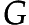with a binary operation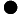, identity element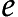and inverse element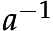for each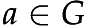. With an obvious laws you can imagine relating these.

If we remove identity element from a group, "obviously" we get a semigroup. Because if there’s any element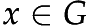, we can recover the identity element by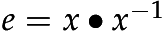.

So what’s a semigroup with identity element? For sometimes it were just that, until we started call it monoid. Go figure, naming is hard, not only in programming, but also in mathematics.

## #Category, Groupoid, Semigroupoid

You are hopefully familiar with a concept of a category. I repeat a definition here:

Definition (Awodey 1.1) A category consist of the following data

• Objects: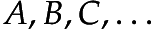• Arrows: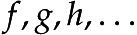• For each arrow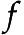, there are given objects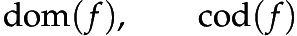called the domain and codomain of. We write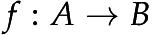to indicate that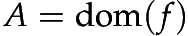and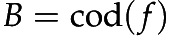.

• Given arrows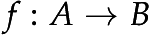and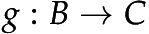, that is, with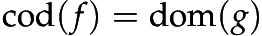there is given an arrow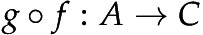called the composite ofand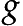.

• For each object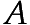, there is given an arrow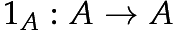called the identity arrow of.

• Associativity: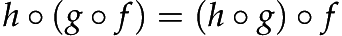for all,,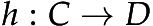.

• Unit: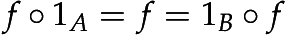for all.

If you think hard (or read a book), you’ll learn that a single object category is a monoid: category arrows are monoid elements, and the laws work out.

The group analogue is called groupoid. In addition to category data, we require that for each arrowthere is an inverse arrow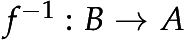, such that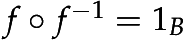and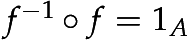. Or more succinctly: that each arrow is an isomorphism.

But we can also remove stuff: if we remove identity arrows, and unit law we get semigroupoid.

Definition A semigroupoid consist of the following data

• Objects:• Arrows:• For each arrow, there are given objectscalled the domain and codomain of. We writeto indicate thatand.

• Given arrowsand, that is, withthere is given an arrowcalled the composite ofand.

• Associativity:for all,,.

A reader probably ask themselves: are there interesting, not-contrived examples of semigroupoids, which aren’t also categories? There are. If poset (set with partial order) is an example of category, then a set with strict order1, is an example of semigroupoid.

As a concrete example, natural numbers with an unique arrow between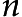and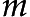when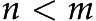is a semigroupoid.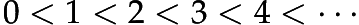There are no identity arrow, as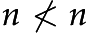, but associativity works out: ifand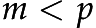then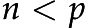. Let’s call this semigroupoid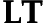.

Finally, a plot twist. nLab calls semigroupoids semicategories, and don’t even mention a semigroupoid as an alternative name!

## #Functors, Semifunctors...

Recall a definition of functor.

Definition (Awodey 1.2) A functor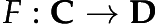between categories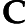and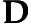is a mapping of objects to objects and arrows to arrows, in such a way that

•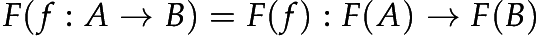,

•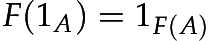,

•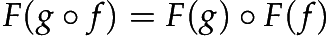.

That is,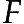preserves domains and codomains, identity arrows, and composition. A functor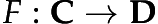thus gives a sort of "picture" – perhaps distorted – ofin.

Functors preserve identities, but semigroupoids don’t have identities to be preserved. We need a weaker concept:

Definition A semifunctorbetween semigroupoidsandis a mapping of objects to objects and arrows to arrows, in such a way that

•,

•.

That is,preserves domains and codomains, and composition. A functorthus gives a sort of "picture" – perhaps distorted – ofin.

An identity functor is obviously a semifunctor, also a successor functor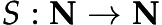is a semifunctor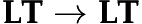.

In Haskell, that would be silly to define a class for (endo)semifunctors:

-- semimap f . semimap g = semimap (f . g)
class Semifunctor f where
semimap :: (a -> b) -> f a -> f b

It’s the Functor type-class without an identity law. On the other hand, something like

data Mag a b t where
Map   :: (x -> t) -> Mag a b x -> Mag a b t
One   :: a -> Mag a b b

instance Semifunctor (Mag a b) where
fmap f (Map g x) = Map (f . g) x
fmap f x         = Map f x

would be valid.

Now as we have semifunctors, would it make sense to ask whether endosemifunctors can form a monad

Semimonad A semimonad on semigroupoid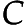consists of

• an endosemifunctor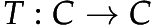• semi natural transformation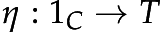(return)

• semi natural transformation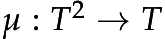(join)

• Associativity (as semi natural transformations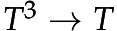)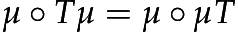• Identity (as semi natural transformations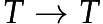)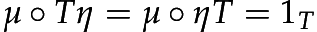Looks a lot like monad, but for semifunctors. I have an example: the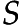semifunctor is a semimonad. Feels like (I didn’t check) that all strictly monotonic functions would fit. We need to find some more structured semigroupoid thanto find more interesting semimonads, but I haven’t yet.

I end with a catchy phrase:

A semimonad is a monoid (!) in the category of endosemifunctors.

What would be a semigroup in the category of endofunctors2, or a semigroup in the category of endosemifunctors?

Naming is hard.

## #References: More on the theory of semi-functors

There’s a paper The theory of semi-functors by Raymond Hoofman https://doi.org/10.1017/S096012950000013X.

1. http://mathworld.wolfram.com/StrictOrder.html, I not dare to call the set with strict order a soset

2. in Haskell we call it Bind, at least now (or Apply for different semigroup)

Site proudly generated by Hakyll